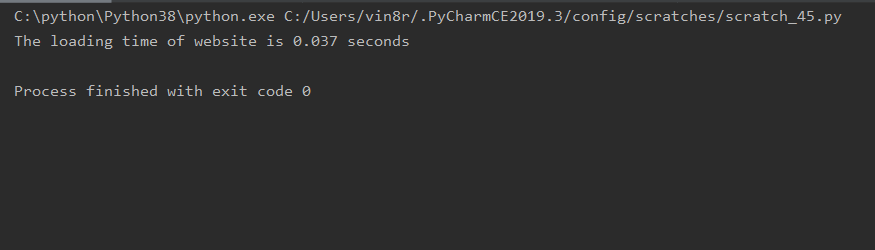# How to Check Loading Time of Website using Python

• Last Updated : 11 Jul, 2022

In this article, we will discuss how we can check the website’s loading time.

Do you want to calculate how much time it will take to load your website? Then, what you must need to exactly do is subtract the time obtained passed since the epoch from the time obtained after reading the whole website.

## Stepwise Implementation:

### Step 1: Import the libraries

In this step, we are simply importing the required libraries to the working python environment to use its functionalities further as per needed.

Here, we are importing the urllib.request and time libraries, the urllib.request library defines functions and classes which help in opening URLs, while the time library defines the time which helps in returning the number of seconds passed since the epoch.

```from urllib.request import urlopen
from time import time```

### Step 2: Obtain the website

In this step, we have used the urlopen() function in which we have to pass the URL of the website as an argument for obtaining the website.

`website = urlopen('#Url of the website')`

### Step 3: Return the time passed since the epoch

In this step, we have used the function time() which will return the number of seconds passed since the epoch.

`open_time = time()`

### Step 4: Read the entire website

In this step, we will use the read() function which will read the contents of the entire file.

`output = website.read()`

### Step 5: Return the time passed since the epoch

In this step, we have used the function time() which will return the number of seconds passed since the epoch.

`close_time = time()`

### Step 6: Close the website

In this step, we have used the close() function that closes the opened file.

`website.close()`

### Step 7: Subtract the time obtained passed since the epoch from the time obtained after reading the whole website

In this step, we will subtract the time obtained passed since the epoch from the time obtained after reading the whole website.

## Python3

 `# Python program to check the``# loading time of the website`` ` `# Importing the libraries``from` `urllib.request ``import` `urlopen``from` `time ``import` `time`` ` `# Obtaining the URL of website``website ``=` `urlopen(``'https://www.geeksforgeeks.org/'``)`` ` `# Return the number of seconds``# passed since epoch``open_time ``=` `time()`` ` `# Read the complete website``output ``=` `website.read()`` ` `# Return the number of seconds ``# passed since epoch``close_time ``=` `time()`` ` `# Close the website``website.close()`` ` `# Subtract and print the open time ``# of website from close time``print``(``'The loading time of website is'``,``round``(close_time``-``open_time,``3``),``'seconds'``)`

### Output:My Personal Notes arrow_drop_up